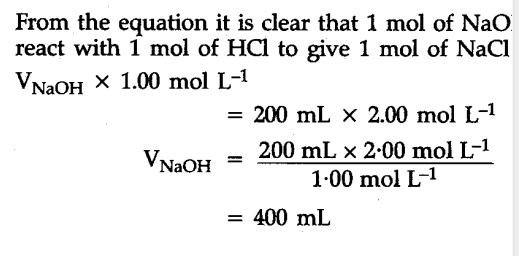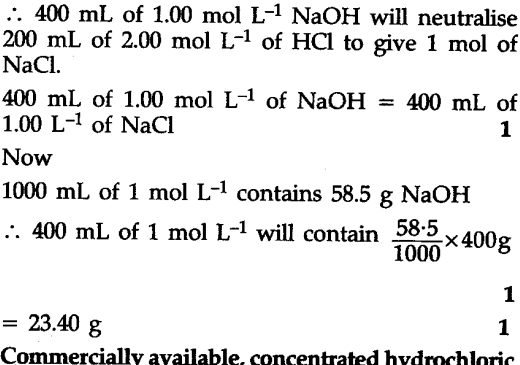# Calculate the volume of 1.00 mol \${{L}^{-1}}\$ aqueous sodium hydroxide that is neutralised by 200 mL of 2.00 mol \${{L}^{-1}}\$ aqueous hydrochloric acid arid the mass of sodium chloride produced

Calculate the volume of 1.00 mol \${{L}^{-1}}\$ aqueous sodium hydroxide that is neutralised by 200 mL of 2.00 mol \${{L}^{-1}}\$ aqueous hydrochloric acid arid the mass of sodium chloride produced.
Neutralization reaction is :
NaOH(aq) + HCl(aq) -> NaCl(aq) + \${{H}_{2}}\$O(I)Thankew soo… Much😁